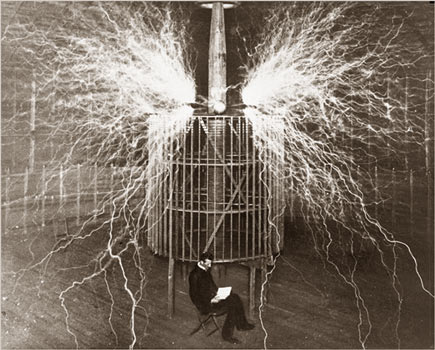return to homepagereturn to updatesMy Unified Field Equationis confirmedby Miles Mathis Abstract: I show that my unified field equation for force, derived several years ago, and my unified field equation for velocity, derived recently to solve the galactic rotation problem, resolve. This confirms both solutions and both papers, as well as my papers on π.In my first paper on the unified field, I derived a relativistic UFT equation in the form F = (GmM/r2) – (2GmM/rct) I did this with simple postulates, and without any curved field, tensors, or other difficult math. In my more recent paper on the galactic rotation problem, I developed a similar equation for the velocity of an orbiter: v = √[(GM0/r) – (Gmr/r)] Question is, can we derive one from the other? In the galactic rotation paper, I didn't use my unified field equation, instead deriving the velocity equation from first postulates again. But we can see from the form that they are related, both being differentials of the same sort. The first thing to do is multiply the first equation by r/m, to achieve ar = (GM/r) – (2GM/ct) Then we take the square root v = √[(GM/r) – (2GM/ct)] If mr/r = 2M/ct, we have a match. We can rewrite that equation as mr/M = 2r/ct That implies that the orbiting mass is dependent on the central mass, something current equations deny. Let us solve for t, to see what time that is. t = 2rM/cmr If we solve for Jupiter, t = 5,466,035s Since that isn't the time for one orbit, we have more work to do. It turns out we are off by a factor of 68 in this time period, which should be a factor of 64. Yes, that is 1/68 of the period of Jupiter. How do I know it should be 64? Because in the equation we are comparing the square root of the time to the velocity. The square root of 64 is 8, so the relationship of the velocity to the time is 8. In another paper, I have shown that the tangent is equal to the radius only when we are at 1/8 of the circle. Therefore, the time here is the time for the orbiter to travel 1/8 of the orbit. Only after that time will the tangent equal the radius, and only at that time will the arc equal the tangent. If the arc equals the tangent and the tangent is defined as the velocity, then we can find the velocity straight from the radius. Since these equalities work only at 1/8 of the circle (rather than at a limit, as was previously thought), the time period in this equation must apply to 1/8 of the circle. You will say we are still off by a factor of 1.06. But as I showed in my paper on the Stefan-Boltzmann Law, that is the difference between 4 and π, at the fourth root. 4√4/4√π = 1.06. Here, we have 8 as the square root of 64, and the time as the square root of the velocity, so we are at the fourth root. You will notice that π doesn't exist in my equations, although my equations can include an orbit. If we want to insert current orbital numbers into my new equations, we have to transform between π and 4. The number 1.06 is the transform here. Therefore, the equations do match. As long as we define t as the time for 1/8 of an orbit, we can derive one equation from the other. This verifies both equations, as well as my jettisoning of π in the kinematic circle. If this paper was useful to you in any way, please consider donating a dollar (or more) to the SAVE THE ARTISTS FOUNDATION. This will allow me to continue writing these "unpublishable" things. Don't be confused by paying Melisa Smith--that is just one of my many noms de plume. If you are a Paypal user, there is no fee; so it might be worth your while to become one. Otherwise they will rob us 33 cents for each transaction.